You have no items in your shopping cart.
Search

# Irrigation Run Time

Use this calculator to determine the length of time that an irrigation system must run to apply enough water to replace the water lost to evapotranspiration. This depends on the irrigation frequency, the water use rate of the crop, the irrigation application rate (precipitation rate), and the irrigation efficiency.

The results are not applicable to self-propelled irrigation systems such as center pivots, linear moves, or hose-reel systems..

## Irrigation Run Time Calculator

Watering Interval:
Crop Water Use:
Irrigation Application Rate:
Irrigation Efficiency:
Irrigation Run Time:

### The Equation

This calculator uses this formula to determine the run time of an irrigation system.Where: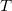= The time the irrigation system needs to run (hrs)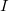= The watering interval of the system (day)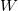= Crop Water Use (in/day)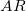= Application Rate of the system (in/hr)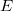= The efficency of the irrigation system (as a decimal)

Reference: Washington State University